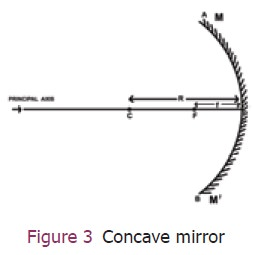Home | | Science 9th std | Curved Mirrors

# Curved Mirrors

We studied about laws of reflection. These laws are applicable to all types of reflecting surfaces including curved surfaces.

Curved Mirrors

We studied about laws of reflection. These laws are applicable to all types of reflecting surfaces including curved surfaces. Let us learn about image formation in curved surfaces in this part.

In your earlier classes, you have studied that there are many types of curved mirrors, such as spherical and parabolic mirrors. The most commonly used type of curved mirror is spherical mirror. The curved surfaces of a shining spoon could also be considered as a curved mirror.

Take a hemispherical spoon. It has an inner and outer surface like the inside and outside of the ball. See your face on these surfaces? How do they look?Move the spoon slowly away from your face.

Observe the image. How does it change?

Reverse the spoon and repeat the activity.

How does the image look like now?

## 1. Spherical mirrors

In curved mirrors, the reflecting surface can be considered to form a part of the surface of a sphere. Such mirrors whose reflecting surfaces are spherical are called spherical mirrors.In some spherical mirrors the reflecting surface is curved inwards, that is, it faces towards the centre of the sphere. It is called concave mirror. In some other mirrors, the reflecting surface is curved outward. It is called convex mirror and are shown in Figure 2.

In order to understand reflection of light at curved surfaces, we need to know the following.

Centre of curvature (C): The centre of the hollow sphere of which the spherical mirror forms a part.

Pole (P): The geometrical centre of the spherical mirror.

Principal axis (PC): The perpendicular line joining the pole and the centre of curvature of the mirror.

Radius of curvature(R): The distance between the pole and the centre of curvature of the spherical mirror.

Principal focus (F): The point on the principal axis of the spherical mirror where the rays of light parallel to the principal axis meet or appear to meet after reflection from the spherical mirror.

Focal length(f): The distance between the pole and the principal focus.Radius of curvature and focal length are related to each other by the formula: R=2f. All these are depicted in Figure 3.

### Check yourself:

·        Focal length of a concave mirror is 5 cm. Find its radius of curvature.

·        For a concave mirror the distance between P and C is 10 cm. Calculate it’s the focal length.

·        A concave mirror has radius of curvature 20 cm. Find the focal length of the mirror.

Tags : Light | Science , 9th Science : Light
Study Material, Lecturing Notes, Assignment, Reference, Wiki description explanation, brief detail
9th Science : Light : Curved Mirrors | Light | Science